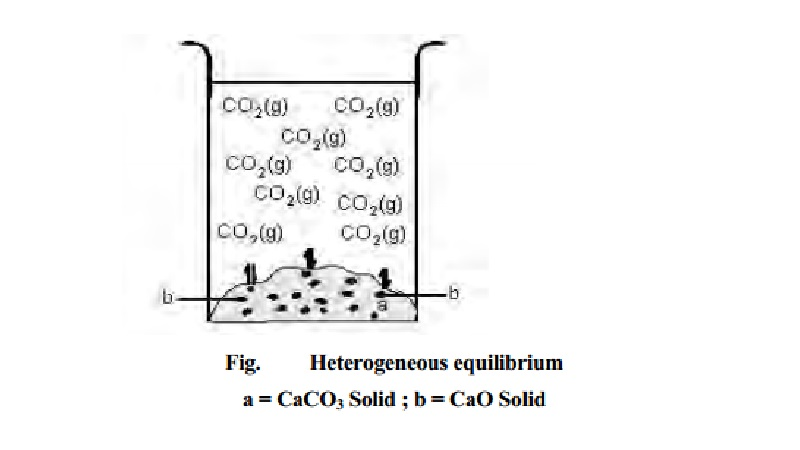Home | | Chemistry | Heterogeneous equilibria

# Heterogeneous equilibriaThe chemical equilibrium in which the reactants and products are not in the same phases are called heterogeneous equilibrium. An example of heterogeneous equilibrium can be the decomposition of calcium carbonate which upon heating forms calcium oxide and carbondioxide under equilibrium conditions.

Heterogeneous equilibria

The chemical equilibrium in which the reactants and products are not in the same phases are called heterogeneous equilibrium. An example of heterogeneous equilibrium can be the decomposition of calcium carbonate which upon heating forms calcium oxide and carbondioxide under equilibrium conditions. When the reaction is carried out in a closed vessel, the following heterogeneous equilibrium is established.

CaCO3    -- > < --   CaOs) + CO2(g)

The equilibrium constant expression for CaCO3  dissociation can be written as

K = [CO2] [CaO] / [CaCO3]

[CaCO3]

But CaO and CaCO3 are pure solids. The activity or concentration of pure solids is unity.

Thus Kc     =    [CO2]

in terms of partial pressures,

Kp = pco2, where Pco2 is the pressure of CO2 alone in equilibrium. There are many examples of heterogeneous chemical equilibria with Kp and Kc values different depending on the number of product and reactant molecules.

(i) The equilibrium constant expression for decomposition of liquid water would be

2H2O(l)  -- > < --- 2H2(g) + O2(g)

K  = [H2]2 [O2] / [H2O]2 but activity of liquid H2O = 1.0

Kc  = [H2]2 [O2]

and Kp  = (pH2)2 (pO2)     p = partial pressure.

(ii) Consider the equilibrium reaction of decomposition of NH4 Cl.

NH4Cl(s) -- > < -- NH3(g) + HCl(g)

Kc = [NH3] [HCl] because [NH4Cl(5)] = 1.0

Kp = PNH3   PHCl.P=Partial Pressure

(iii) Consider the hydrogen gas evolution equilibrium such as

3Fe(s) + 4H2O(g) -- > < -- Fe3O4   +   4H2(g)

Kc = [H2]4 / [H2O]4

kp  = (pH2)2 / (pH2O)4

since activities of Fe(G) and Fe3O4(s) are 1.0.Study Material, Lecturing Notes, Assignment, Reference, Wiki description explanation, brief detail
11th 12th std standard Class Organic Inorganic Physical Chemistry Higher secondary school College Notes : Heterogeneous equilibria |